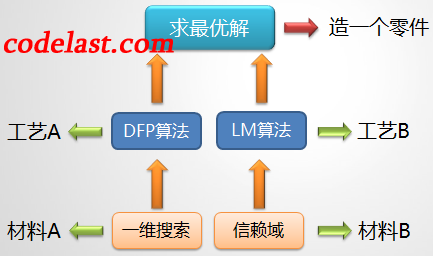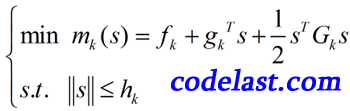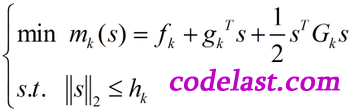【1】信赖域算法与一维搜索算法的区别、联系【2】信赖域算法的基本思想

【3】信赖域算法的数学模型$k$ 次迭代的实际下降量为： $\Delta {f_k} = {f_k} - f({x_k} + {s_k})$
$k$ 次迭代的预测下降量为： $\Delta {m_k} = {f_k} - m({s_k})$

【4】信赖域算法的步骤

• 从初始点 ${x_0}$ ，初始信赖域半径 ${h_0} = \left\| {{g_0}} \right\|$ 开始迭代
• 到第 $k$ 步时，计算 ${g_k}$${G_k}$
• 解信赖域模型，求出位移 ${s_k}$ ，计算 ${r_k}$
• ${r_k} \le 0.25$ ，说明步子迈得太大了，应缩小信赖域半径，令 ${h_{k + 1}} = \frac{{\left\| {{s_k}} \right\|}}{4}$
• ${r_k} \ge 0.75$$\left\| {{s_k}} \right\| = {h_k}$ ，说明这一步已经迈到了信赖域半径的边缘，并且步子有点小，可以尝试扩大信赖域半径，令 ${h_{k + 1}} = 2{h_k}$
• $0.25 < {r_k} < 0.75$ ，说明这一步迈出去之后，处于“可信赖”和“不可信赖”之间，可以维持当前的信赖域半径，令 ${h_{k + 1}} = {h_k}$
• ${r_k} \le 0$ ，说明函数值是向着上升而非下降的趋势变化了（与最优化的目标相反），这说明这一步迈得错得“离谱”了，这时不应该走到下一点，而应“原地踏步”，即 ${x_{k + 1}} = {x_k}$ ，并且和上面 ${r_k} \le 0.25$ 的情况一样缩小信赖域。反之，在 ${r_k} > 0$ 的情况下，都可以走到下一点，即 ${x_{k + 1}} = {x_k} + {s_k}$

【5】最重要的一种信赖域算法：Levenberg-Marquardt算法【6】信赖域算法的收敛性

[原创]信赖域(Trust Region)算法是怎么一回事

### 4 thoughts on “[原创]信赖域(Trust Region)算法是怎么一回事”

• 2016 年 12 月 21 日 at 19:58
Permalink

“若 rk≤0.25 ，说明步子迈得太大了，应缩小信赖域半径” 这种情况应该是预测的函数下降的比实际函数大吧，这时要缩小信頼域半径，但是前面有一句“若不能使目标函数值充分下降，则缩小信赖域”，感觉这里是不是矛盾？

Reply
• 2017 年 05 月 06 日 at 18:04
Permalink

我也觉得可能是写反了，刚开始学习，并不太懂哈哈还是傻瓜的声援你一下，说不定作者看到会解释

Reply
• 2017 年 11 月 27 日 at 09:03
Permalink

我觉得并不是矛盾。预测的下降比实际的下降大很多，说明你预测的太远了，要收回来。所以要缩小信赖域半径。这里的rk<=0.25不就是“不能使目标函数值充分下降”的体现吗？

Reply
• 2018 年 02 月 26 日 at 14:54
Permalink

Rk1表示预测比实际下降的快，信赖域要减小

Reply